# 一文学会动态规划解题技巧

danielmou

0收藏

• 什么是动态规划
• 动态规划从入门到进阶
• 再谈动态规划

### 什么是动态规划

1. 多阶段决策，意味着问题可以分解成子问题，子子问题，。。。，也就是说问题可以拆分成多个子问题进行求解
2. 最优子结构，在自下而上的递推过程中，我们求得的每个子问题一定是全局最优解，既然它分解的子问题是全局最优解，那么依赖于它们解的原问题自然也是全局最优解。
3. 自下而上，怎样才能自下而上的求出每个子问题的最优解呢，可以肯定子问题之间是有一定联系的，即迭代递推公式，也叫「状态转移方程」，要定义好这个状态转移方程， 我们就需要定义好每个子问题的状态（DP 状态），那为啥要自下而上地求解呢，因为如果采用像递归这样自顶向下的求解方式，子问题之间可能存在大量的重叠，大量地重叠子问题意味着大量地重复计算，这样时间复杂度很可能呈指数级上升（在下文中我们会看到多个这样重复的计算导致的指数级的时间复杂度），所以自下而上的求解方式可以消除重叠子问题。

1. 判断是否可用递归来解，可以的话进入步骤 2
2. 分析在递归的过程中是否存在大量的重复子问题
3. 采用备忘录的方式来存子问题的解以避免大量的重复计算（剪枝）
4. 改用自底向上的方式来递推，即动态规划

### 动态规划从入门到进阶

#### 入门题：斐波那契数列

1、判断是否可用递归来解 显然是可以的，递归代码如下

``````    public     static     int fibonacci(    int{
if (n == 1)     return1;
if (n == 2)     return2;
return fibonacci(n - 1) + fibonacci(n - 2);
}``````

2、分析在递归的过程中是否存在大量的重复子问题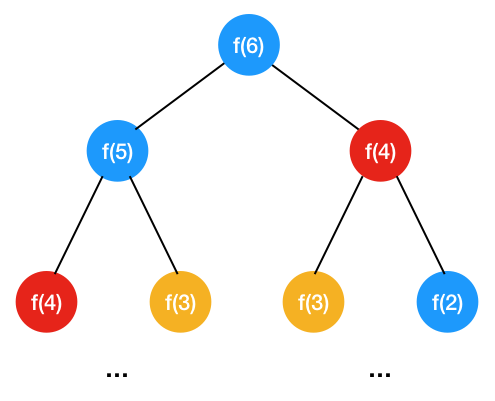3、采用备忘录的方式来存子问题的解以避免大量的重复计算 既然以上中间子问题中存在着大量的重复计算，那么我们可以把这些中间结果给缓存住（可以用哈希表缓存），如下

``````    public     static     int fibonacci(    int{
if (n = 1)     return1;
if (n = 2)     return2;
if (map.get(n) !=     null)  {
return map.get(n);
}
int result = fibonacci(n - 1) + fibonacci(n - 2);
map.put(n, result);
return result;
}``````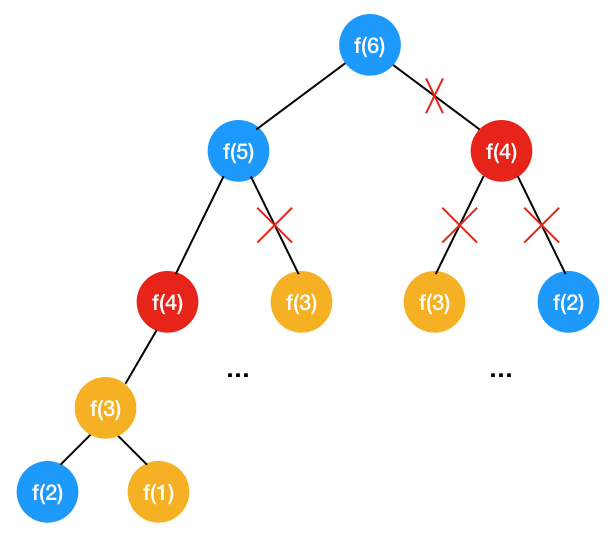4、改用自底向上的方式来递推，即动态规划 我们注意到如下规律

``````f(1) = 1
f(2) = 2
f(3) = f(1) + f(2) = 3
f(4) = f(3) + f(2) = 5
....
f(n) = f(n-1) + f(n-2)``````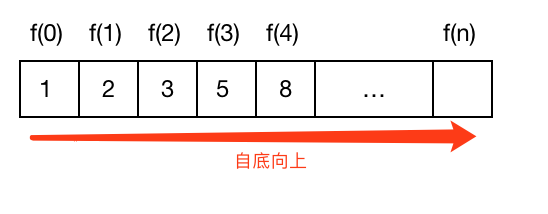f(n) 就是定义的每个子问题的状态（DP 状态），f(n) = f(n-1) + f(n-2) 就是状态转移方程，即 f(n) 由 f(n-1), f(n-2) 这两个状态转移而来,由于每个子问题只与它前面的两个状态，所以我们只要定义三个变量，自底向上不断循环迭代即可，如下

``````    public     int f(    int{
if (n == 1)     return1;
if (n == 2)     return2;
int result = 0;
int pre = 1;
int next = 2;

for (    int i = 3; i < n + 1; i ++) {
result = pre + next;
pre = next;
next = result;
}
return result;
}``````

#### 小试牛刀：三角形的最小路径和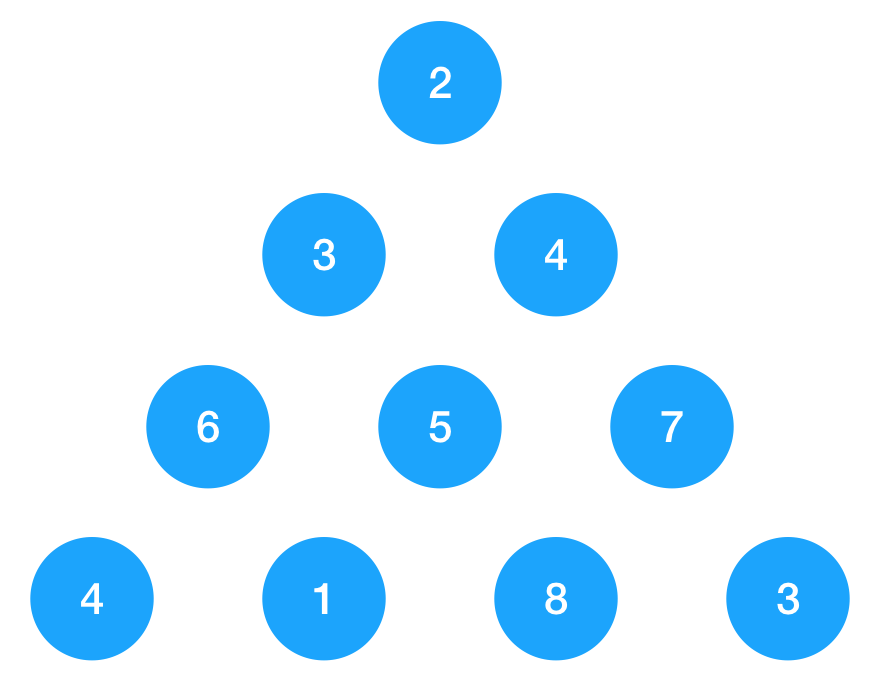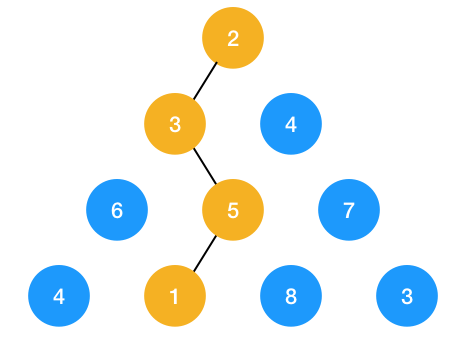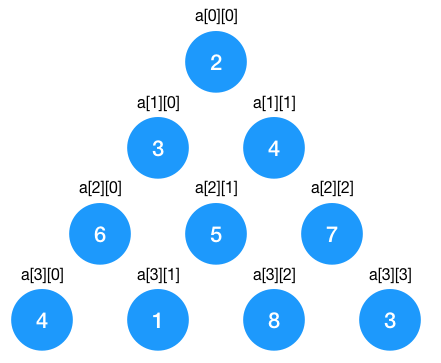1、 判断是否可用递归来解

``````traverse(i, j) = {
traverse(i+1, j);    向节点i,j 下面的左节点走一步
traverse(i+1, j+1);    向节点i,j 下面的右节点走一步
}``````

``````    private    static    int[][] triangle = {
{2, 0, 0, 0},
{3, 4, 0, 0},
{6, 5, 7, 0},
{4, 1, 8, 3}
};

public     static     int traverse(    int i,     int{
int totalRow = 4; // 总行数
if (i >=  totalRow - 1) {
return0;
}
// 往左下节点走时
int leftSum = traverse(i+1, j) + triangle[i+1][j];
// 往右下节点走时
int rightSum = traverse(i+1, j+1) + triangle[i+1][j+1];
// 记录每个节点往左和往右遍历的路径和的最小值
return Math.min(leftSum, rightSum);
}

public     static      void main(String[] args)      throws{
int sum = traverse(0, 0) + triangle;
System.out.println("sum = " + sum);
}``````

``````traverse(i, j) = {
traverse(i+1, j);    向节点i,j 下面的左节点走一步
traverse(i+1, j+1);    向节点i,j 下面的右节点走一步
}``````

2、分析在递归的过程中是否存在大量的重复子问题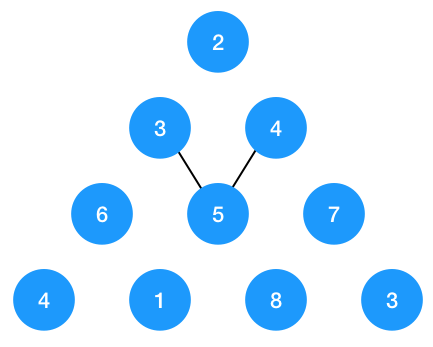3、采用备忘录的方式来存子问题的解以避免大量的重复计算（剪枝）

``````    private    static    int[][] triangle = {
{2, 0, 0, 0},
{3, 4, 0, 0},
{6, 5, 7, 0},
{4, 1, 8, 3}
};

// 记录中间状态的 map
private    static HashMap<String, Integer> map =     new HashMap();

public     static     int traverse(    int i,     int{
String key = i + "" + j;
if (map.get(key) !=     null) {
return map.get(key);
}

int totalRow = 4; // 总行数
if (i >=  totalRow - 1) {
return0;
}
// 往左下节点走时
int leftSum = traverse(i+1, j) + triangle[i+1][j];
// 往右下节点走时
int rightSum = traverse(i+1, j+1) + triangle[i+1][j+1];
// 记录每个节点往左和往右遍历的路径和的最小值
int result = Math.min(leftSum, rightSum);
map.put(key, result);
return result;
}``````

4、改用自底向上的方式来递推，即动态规划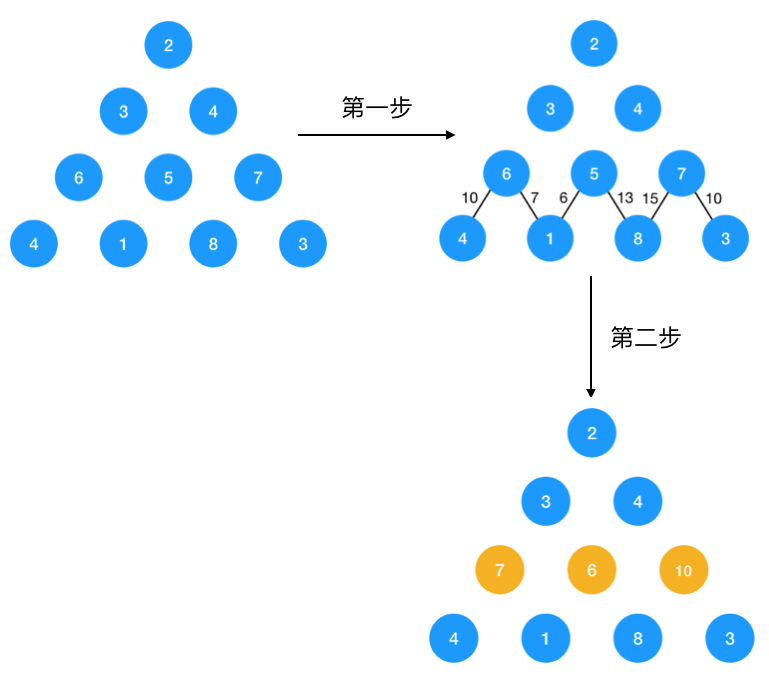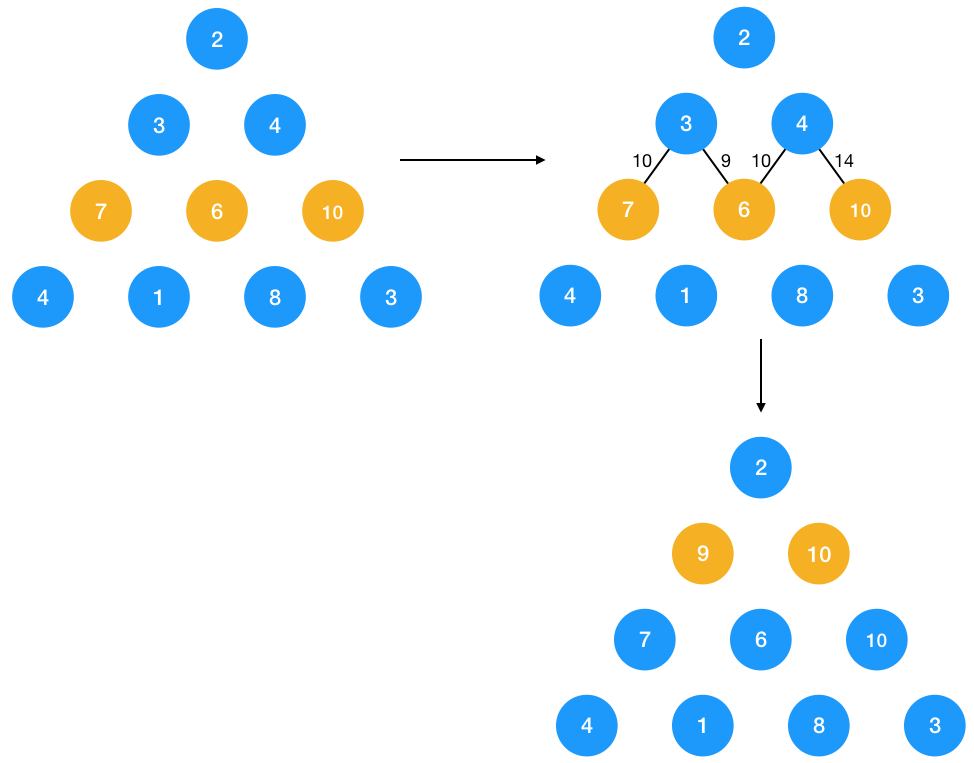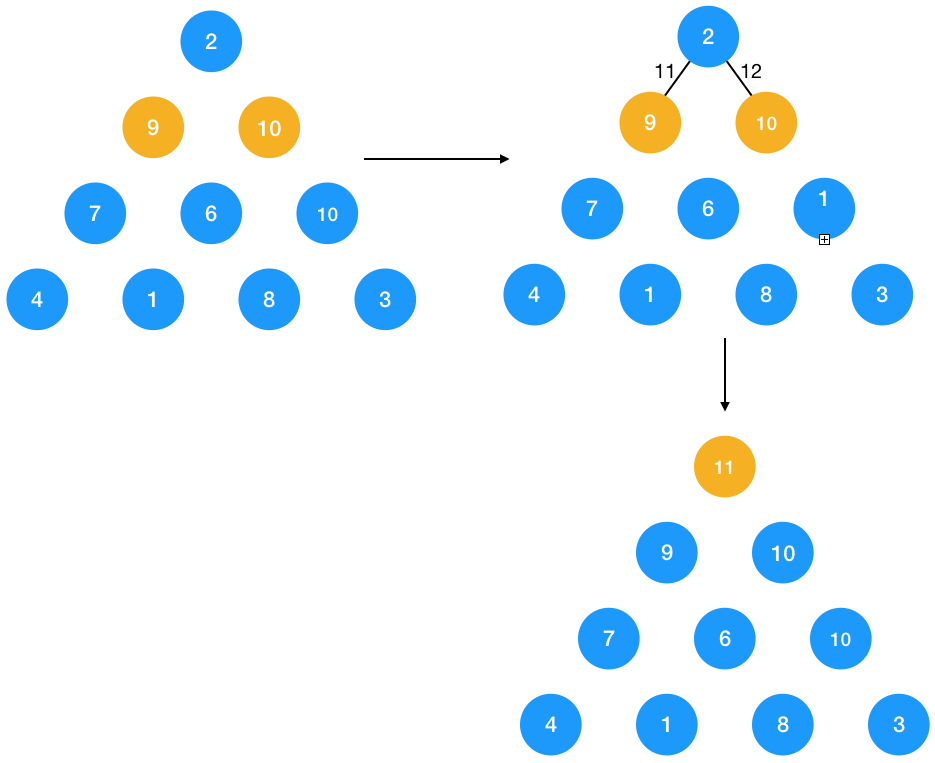``DP[i,j] = min(DP[i+1, j], D[i+1, j+1]) + triangle[i,j]``

DP 状态 DP[i,j] 有两个变量，需要分别从下而上，从左到右循环求出所有的 i,j, 有了状态转移方程求出代码就比较简单了，如下

``````    private    static    int[][] triangle = {
{2, 0, 0, 0},
{3, 4, 0, 0},
{6, 5, 7, 0},
{4, 1, 8, 3}
};
public     static     int traverse(){
int ROW = 4;
int[] mini = triangle[ROW - 1];
// 从倒数第二行求起，因为最后一行的值本身是固定的
for (    int i = ROW - 2; i >= 0; i--) {
for (    int j = 0; j < triangle[j].length; j++) {
mini[j] = triangle[i][j] + Math.min(mini[j], mini[j+1]);
}
}
return mini;
}

public     static      void main(String[] args)      throws{
int minPathSum = traverse();
System.out.println("sum = " + minPathSum);
}``````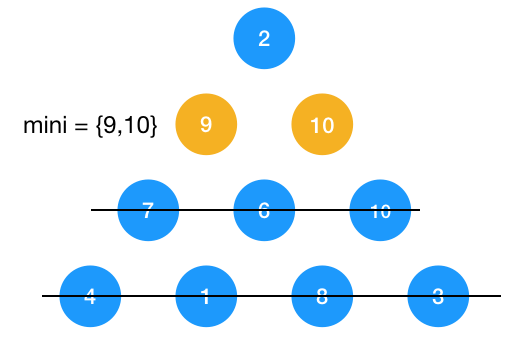#### 进阶：凑零钱

1、定义这个函数，明确这个函数的功能,函数的功能显然是给定一个 amount，用定义好的 coins 来凑，于是我们定义函数如下

``````    private     static     int f(    int amount,     int[] coins){
}``````

2、寻找问题与子问题的关系，即递推公式 这题的递推关系比较难推导，我们一起看下，假设 f(amount, coins) 为零钱 amount 的所需要的最少硬币数，当选中了coins 中的第一枚硬币之后（即为 coins），则需再对剩余的 amount - coins 金额求最少硬币数，即调用 f(amount - coins, coins)  ,由此可知当选了第一枚硬币后的递推公式如下

``f(amount, coins) = f(amount-coins) + 1 (这里的 1 代表选择了第一枚硬币)``

``````f(amount, coins) = f(amount-coins) + 1 (这里的 1 代表选择了第二枚硬币)
f(amount, coins) = f(amount-coins) + 1 (这里的 1 代表选择了第三枚硬币)``````

``f(amount, coins) = min{ f(amount - coins[i]) + 1) }, 其中 i 的取值为 0 到 coins 的大小，coins[i] 表示选择了此硬币, 1 表示选择了coins[i]  这一枚硬币``

3、将第二步的递推公式用代码表示出来补充到步骤 1 定义的函数中

``````    public    class Solution {

private     static     int exchange(    int amount,     int[] coins){

// 说明零钱刚好凑完
if (amount == 0) {
return0;
}

// 说明没有满足的条件
if (amount < 0) {
return -1;
}

int result = Integer.MAX_VALUE;
for (    int i = 0; i < coins.length; i++) {
int subMin = exchange(amount - coins[i], coins);
if (subMin == -1)     continue;
result = Math.min(subMin + 1, result);
}

// 说明没有符合问题的解
if (result == Integer.MAX_VALUE) {
return -1;
}

return result;
}

public     static      void main(String[] args)      throws{
int amount = 11;
int[] coins = {1,2,5};
int result = exchange(amount, coins);
System.out.println("result = " + result);
}
}``````

4、计算时间复杂度 这道题的时间复杂度很难看出来，一般看不出来的时候我们可以画递归树来分析，针对 amount = 11 的递归树 如下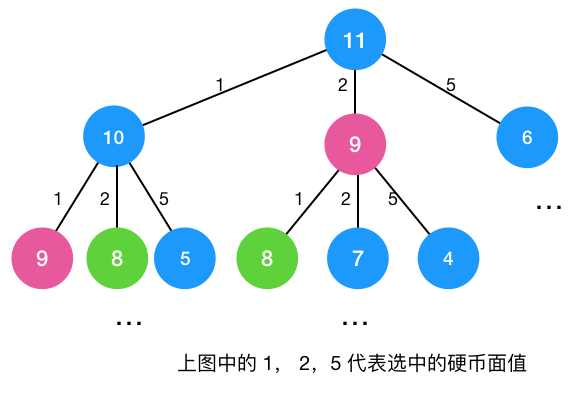``````    public    class Solution {

// 保存中间结果
private    static HashMap<Integer, Integer> map =     new HashMap();

// 带备忘录的递归求解
private     static     int exchangeRecursive(    int amount,     int[] coins){
if (map.get(amount) !=     null) {
return map.get(amount);
}
// 说明零钱已经凑完
if (amount == 0) {
return0;
}

// 说明没有满足的条件
if (amount < 0) {
return -1;
}

int result = Integer.MAX_VALUE;
for (    int i = 0; i < coins.length; i++) {
int subMin = exchangeRecursive(amount - coins[i], coins);
if (subMin == -1)     continue;
result = Math.min(subMin + 1, result);
}

// 说明没有符合问题的解
if (result == Integer.MAX_VALUE) {
return -1;
}

map.put(amount, result);
return result;
}

public     static      void main(String[] args)      throws{
int amount = 11;
int[] coins = {1,2,5};
int result = exchangeRecursive(amount, coins);
System.out.println("result = " + result);
}
}``````

``f(amount, coins) = min{ f(amount - coins[i]) + 1) }, 其中 i 的取值为 0 到 coins 的大小，coins[i] 表示选择了此硬币, 1 表示选择了coins[i]  这一枚硬币``

``DP[i] =  min{ DP[ i - coins[j] ] + 1 } = min{ DP[ i - coins[j] ]} + 1,  其中 j 的取值为 0 到 coins 的大小，i 代表取了 coins[j] 这一枚硬币。``

``````// 动态规划求解
private     static     int exchangeDP(    int amount,     int[] coins){
int[] dp =     new    int[amount + 1];
// 初始化每个值为 amount+1，这样当最终求得的 dp[amount] 为 amount+1 时，说明问题无解
for (    int i = 0; i < amount + 1; i++) {
dp[i] = amount + 1;
}

// 0 硬币本来就没有，所以设置成 0
dp = 0;

for (    int i = 0; i < amount + 1; i++) {
for (    int j = 0; j < coins.length; j++) {
if (i >= coins[j]) {
dp[i] = Math.min(dp[i- coins[j]], dp[i]) + 1;
}
}
}

if (dp[amount] == amount + 1) {
return -1;
}
return dp[amount];
}``````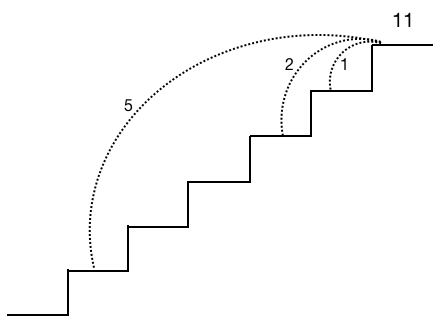`` f(n) = min{ f(n-1)，f(n-2)，f(n-5)} + 1 （1代表最后一跳）``

### 总结帖子
视频
声望
粉丝
相关问题
社区精华内容